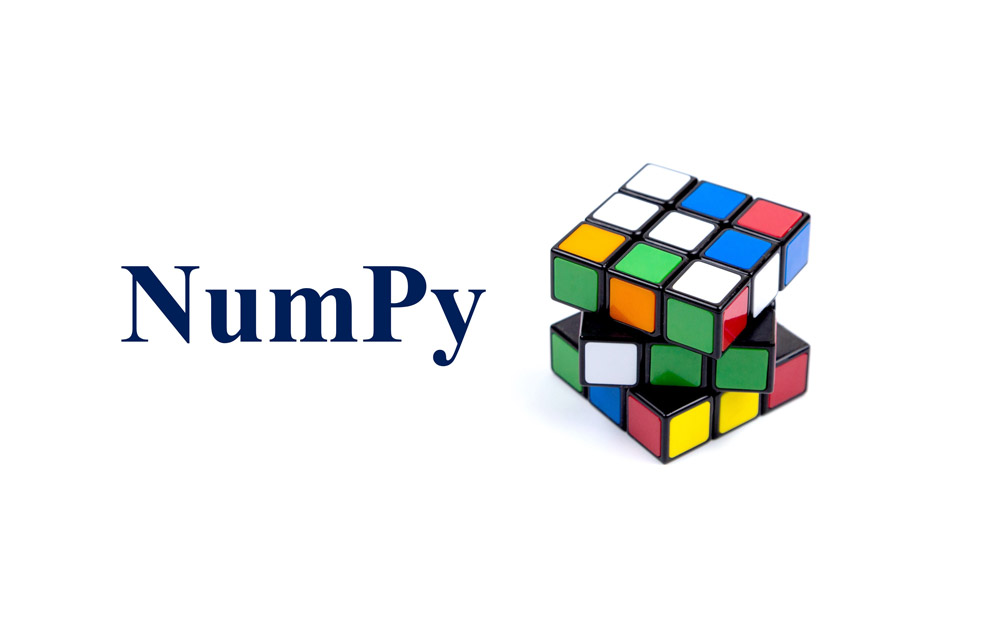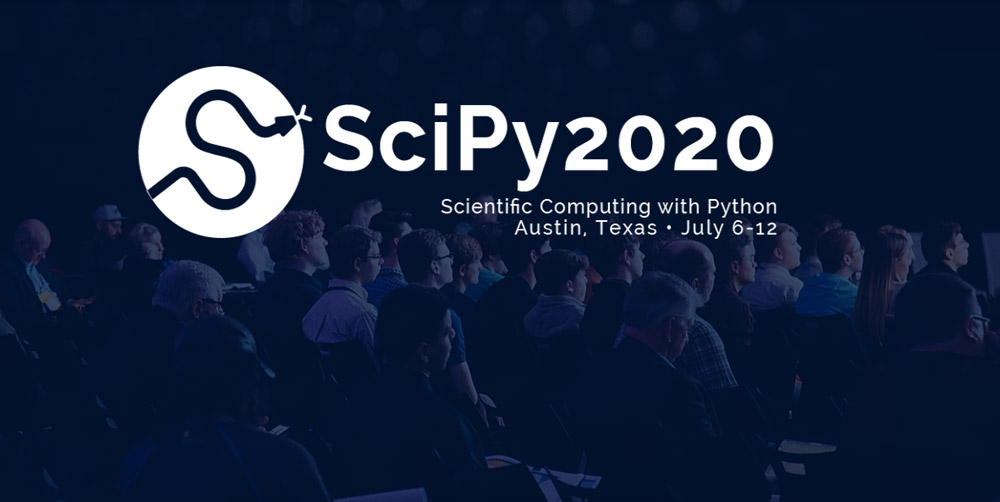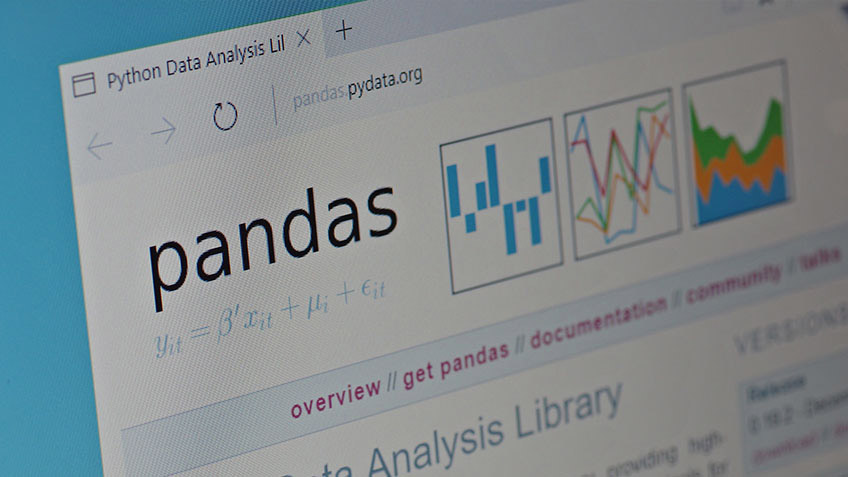# blog posts# Python has a special place among most programming languages . Python is one of the most popular languages ​​developed by data scientists and software developers alike for data science work.

The Python can be used to predict results, automate tasks, simplify processes, and provide business intelligence insights. Follow us in the article Python Libraries of Data Science .

Working with data in Python vanilla is very simple and possible, but we should not forget that there are a few open-source libraries that make Python data work much easier.

### Familiarity with Python libraries

Python is the most widely used programming language today. When it comes to solving the tasks and challenges of data science, Python never stops to surprise its users.

Most data scientists use the power of Python programming every day. Python is an easy-to-learn, debugging, versatile, object-oriented, open source, and و language with many benefits. Python is built with extraordinary libraries that are used every day by programmers to solve problems.

Now if you agree, we go to the best Python science libraries, which are:

#### NumPy

NumPy library is the best option if you want to do computer calculations in Python. NumPy is the first choice among developers and scientists working on Python data. NumPy is registered under the BSD license.

With NumPy you can use a variety of multidimensional array objects, C, C ++, Fortran-based integration tools, functions for complex mathematical operations such as Fourier transform, linear algebra, random number, and more. NumPy is installed under TensorFlow and other sophisticated machine learning operating systems that power their internal activities.Note: The NumPy (Numerical Python) is a convenient tool for calculating and performing basic and advanced array operations.

Things that can be done with the NumPy include:

• Basic Array Operations: Add, Multiply, Cut, Flatten, Deform, Index Arrays
• Work with DateTime or linear algebra.
• Basic cutting and advanced indexing in NumPy Python

#### SciPy

Another Python library of data science that performs scientific calculations is known as SciPy. In a way, SciPy is based on NumPy and extends the capabilities of NumPy. The main structure of SciPy has two multidimensional arrays implemented by Numpy. This library includes tools that help solve linear algebra, probability theory, integral calculus, and much more.Note: SciPy is pronounced as: Sigh Pie

We can see how Python programming helps data scientists in compiling and analyzing large, unstructured datasets such as the TensorFlow, SciKit-Learn, and Eli5 libraries.

The important point is that you should never confuse SciPy with SciPy Stack because the two are completely different. We can use SciPy whenever we want to do things like linear algebra, integration, arithmetic, ordinary differential equations and signal processing.

#### PANDAS

Another popular Python library is Python data analysis. Pandas is an open-source library. Pandas can analyze data structures with high efficiency. We can only use Pandas when We want to make changes to the data structure, which will be much faster and more efficient using this Pandas library.What Pandas does is put the data in a folder called CSV or TSV and put it in a folder called SQL, which is inside other Python libraries that have specific rows and columns. The data frame is very similar to a statistical software table such as Excel or SPSS.

Things to do with Pandas include:

• Indexing, data manipulation, renaming, sorting, merging data frames
• Update or add and remove columns from a data frame.
• Check for lost data or NAN.
• Draw the data with a histogram or box.

Note: Pandas, as a basic library, play an important role in learning Python data science.

#### PyBrain

PyTorch is a framework for scientists who want to do deep learning easily and seamlessly. This tool allows you to perform tensor calculations with GPU acceleration and is also used for other Python tasks.

Create dynamic calculation charts with PyTorch and calculate slopes automatically. Above all, PyTorch is a rich API that helps you solve neural network applications.

PyTorch is based on Torch, which is an open-source deep learning library run in C in Lua. Python API was introduced in 2017, which became very popular over time.

#### TensorFlow

TensorFlow is a popular machine learning and in-depth learning framework developed by Google Brain. This library has aids such as (tflearn, tf-slim, skflow) that make it more useful. TensorFlow is expanding and updating every day. One of the advantages of TensorFlow is that it can fix problems such as security vulnerabilities, improve TensorFlow and GPU integration.

For example, you can run the Estimator model on multiple GPUs on one device. This open source library is designed by Google to calculate data graphs with powerful machine learning algorithms. You may be interested to know that the majority of advanced websites such as Google, Coca-Cola, Airbnb, Twitter, Intel DeepMind, all use TensorFlow.Things to do with TensorFlow include:

• Voice recognition, internet, car, security, UX / UI, telecommunications
• Further analysis for CRM or CX
• Text-based applications, threat detection, Google Translate, Gmail Smart Response
• In-depth Facebook detection, photo tagging, smart unlock
• Movie detection – motion detection, real-time threat detection in games, airports

#### Keras

Keras is a great library for building neural networks and modeling. It is very easy to use and provides good scalability for developers. This library uses other packages (Theano or TensorFlow) as backup.

In addition, Microsoft merged CNTK (Microsoft Cognitive Toolbox) to serve as another backup for the library. If you want to do a quick test using compact systems, Keras is a great option.

Keras is one of the most powerful Python libraries of science . Finally, we hope you find the Python Library data science article useful.